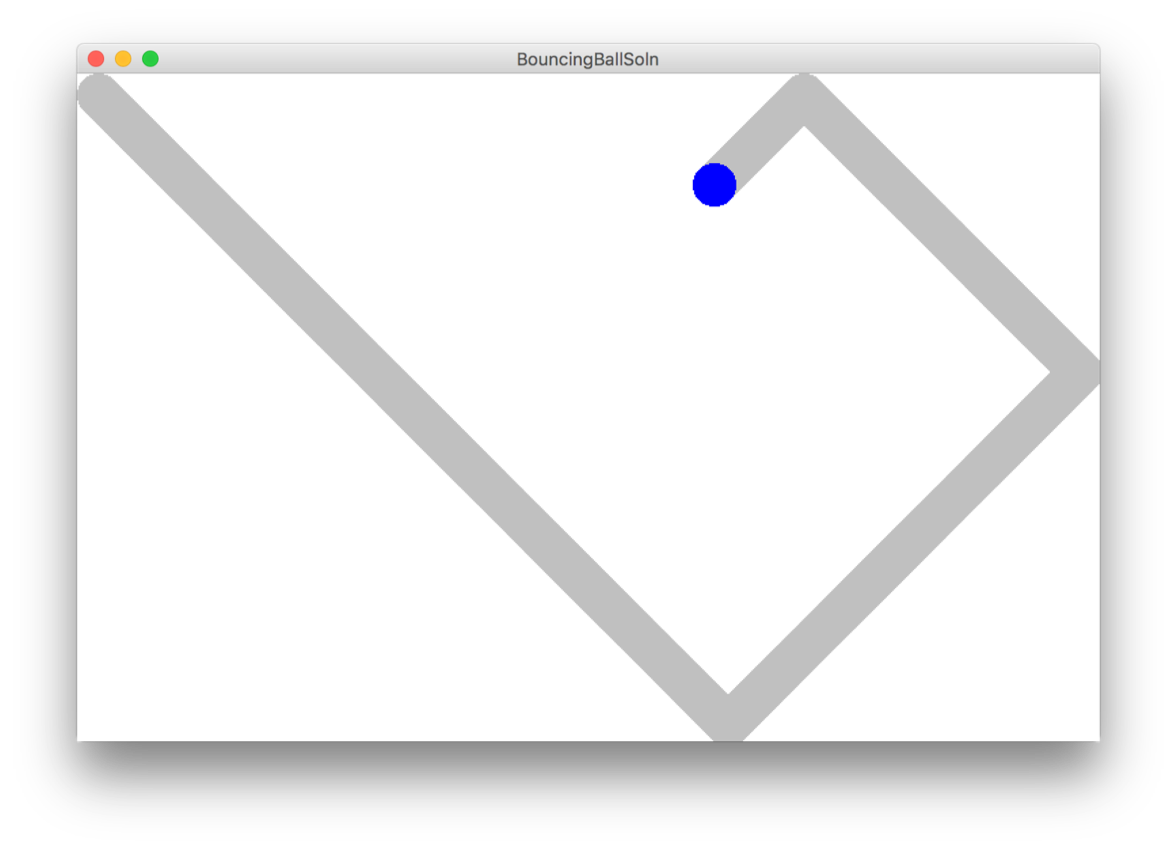# Bouncing Ball

Written by Chris Piech. Many a coder has bounced balls around screens before...

What joy and beauty there can be found in the humble ball bouncing around the screen.## Solution

import tkinter
import time

CANVAS_WIDTH = 600      # Width of drawing canvas in pixels
CANVAS_HEIGHT = 600     # Height of drawing canvas in pixels
CHANGE_X_START = 10
CHANGE_Y_START = 7
BALL_SIZE = 70

def main():
canvas = make_canvas(CANVAS_WIDTH, CANVAS_HEIGHT, 'Bouncing Ball')
ball = canvas.create_oval(0, 0, BALL_SIZE, BALL_SIZE, fill='blue', outline='blue')
change_x = CHANGE_X_START
change_y = CHANGE_Y_START
while True:
# update world
canvas.move(ball, change_x, change_y)
if hit_left_wall(canvas, ball) or hit_right_wall(canvas, ball):
change_x *= -1
if hit_top_wall(canvas, ball) or hit_bottom_wall(canvas, ball):
change_y *= -1
# redraw canvas
canvas.update()
# pause
time.sleep(1/50.)

def hit_left_wall(canvas, object):
return get_left_x(canvas, object) <= 0

def hit_top_wall(canvas, object):
return get_top_y(canvas, object) <= 0

def hit_right_wall(canvas, object):
return get_right_x(canvas, object) >= CANVAS_WIDTH

def hit_bottom_wall(canvas, object):
return get_bottom_y(canvas, object) >= CANVAS_HEIGHT

######## These helper methods use "lists" ###########
### Which is a concept you will learn Monday ###########

def get_left_x(canvas, object):
return canvas.coords(object)

def get_top_y(canvas, object):
return canvas.coords(object)

def get_right_x(canvas, object):
return canvas.coords(object)

def get_bottom_y(canvas, object):
return canvas.coords(object)

######## DO NOT MODIFY ANY CODE BELOW THIS LINE ###########

# This function is provided to you and should not be modified.
# It creates a window that contains a drawing canvas that you
# will use to make your drawings.
def make_canvas(width, height, title):
"""
DO NOT MODIFY
Creates and returns a drawing canvas
of the given int size with a blue border,
reachange_y for drawing.
"""
top = tkinter.Tk()
top.minsize(width=width, height=height)
top.title(title)
canvas = tkinter.Canvas(top, width=width + 1, height=height + 1)
canvas.pack()
return canvas

if __name__ == '__main__':
main()